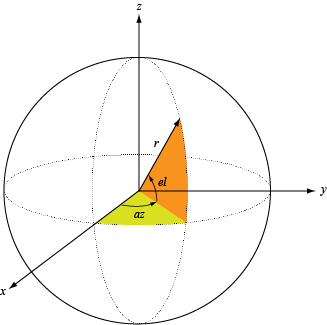Documentation

# directivity

Directivity of antenna or transducer element

## Syntax

``D = directivity(element,FREQ,ANGLE)``

## Description

````D = directivity(element,FREQ,ANGLE)` returns the Directivity of the antenna or transducer element, `element`, at frequencies specified by FREQ in direction angles specified by `ANGLE`.```

## Input Arguments

collapse all

Antenna or transducer element, specified as a Phased Array System Toolbox System object.

Frequencies for computing directivity and patterns, specified as a positive scalar or 1-by-L real-valued row vector. Frequency units are in hertz.

• For an antenna, microphone, or sonar hydrophone or projector element, `FREQ` must lie within the range of values specified by the `FrequencyRange` or `FrequencyVector` property of the element. Otherwise, the element produces no response and the directivity is returned as `–Inf`. Most elements use the `FrequencyRange` property except for `phased.CustomAntennaElement` and `phased.CustomMicrophoneElement`, which use the `FrequencyVector` property.

• For an array of elements, `FREQ` must lie within the frequency range of the elements that make up the array. Otherwise, the array produces no response and the directivity is returned as `–Inf`.

Example: `[1e8 2e6]`

Data Types: `double`

Angles for computing directivity, specified as a 1-by-M real-valued row vector or a 2-by-M real-valued matrix, where M is the number of angular directions. Angle units are in degrees. If `ANGLE` is a 2-by-M matrix, then each column specifies a direction in azimuth and elevation, `[az;el]`. The azimuth angle must lie between –180° and 180°. The elevation angle must lie between –90° and 90°.

If `ANGLE` is a 1-by-M vector, then each entry represents an azimuth angle, with the elevation angle assumed to be zero.

The azimuth angle is the angle between the x-axis and the projection of the direction vector onto the xy plane. This angle is positive when measured from the x-axis toward the y-axis. The elevation angle is the angle between the direction vector and xy plane. This angle is positive when measured towards the z-axis. See Azimuth and Elevation Angles.

Example: `[45 60; 0 10]`

Data Types: `double`

## Output Arguments

collapse all

Directivity, returned as an M-by-L matrix. Each row corresponds to one of the M angles specified by `ANGLE`. Each column corresponds to one of the L frequency values specified in `FREQ`. Directivity units are in dBi where dBi is defined as the gain of an element relative to an isotropic radiator.

collapse all

### Directivity

Directivity describes the directionality of the radiation pattern of a sensor element or array of sensor elements.

Higher directivity is desired when you want to transmit more radiation in a specific direction. Directivity is the ratio of the transmitted radiant intensity in a specified direction to the radiant intensity transmitted by an isotropic radiator with the same total transmitted power

`$D=4\pi \frac{{U}_{\text{rad}}\left(\theta ,\phi \right)}{{P}_{\text{total}}}$`

where Urad(θ,φ) is the radiant intensity of a transmitter in the direction (θ,φ) and Ptotal is the total power transmitted by an isotropic radiator. For a receiving element or array, directivity measures the sensitivity toward radiation arriving from a specific direction. The principle of reciprocity shows that the directivity of an element or array used for reception equals the directivity of the same element or array used for transmission. When converted to decibels, the directivity is denoted as dBi. For information on directivity, read the notes on Element Directivity and Array Directivity.

Computing directivity requires integrating the far-field transmitted radiant intensity over all directions in space to obtain the total transmitted power. There is a difference between how that integration is performed when Antenna Toolbox™ antennas are used in a phased array and when Phased Array System Toolbox antennas are used. When an array contains Antenna Toolbox antennas, the directivity computation is performed using a triangular mesh created from 500 regularly spaced points over a sphere. For Phased Array System Toolbox antennas, the integration uses a uniform rectangular mesh of points spaced 1° apart in azimuth and elevation over a sphere. There may be significant differences in computed directivity, especially for large arrays.

### Azimuth and Elevation Angles

This section describes the convention used to define azimuth and elevation angles.

The azimuth angle of a vector is the angle between the x-axis and its orthogonal projection onto the xy-plane. The angle is positive when going from the x-axis toward the y-axis. Azimuth angles lie between –180° and 180° degrees, inclusive. The elevation angle is the angle between the vector and its orthogonal projection onto the xy-plane. The angle is positive when going toward the positive z-axis from the xy-plane. Elevation angles lie between –90° and 90° degrees, inclusive.